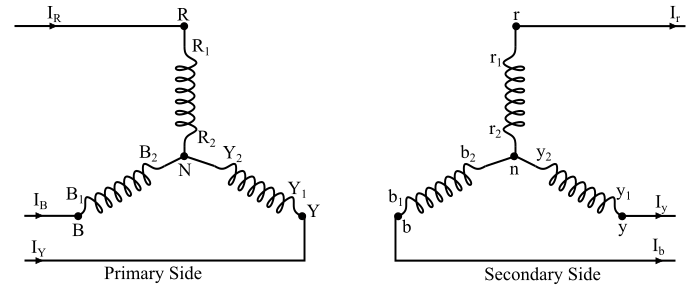# Star-Star Connection of Transformer | Three - Phase Transformer Connection

The star-star connection of transformer is shown in the figure. Here, the one terminal of three windings on each of the primary and the secondary sides being connected at a common point (called neutral point) and the other end of each winding is taken out as the line terminal.

In the case of star-star connection, the phase current is equal to the line current and they are in phase. The line voltage is √3 times of the phase voltage. Also, there is a phase difference of 30° between the line voltages and phase voltages.For an ideal transformer the voltage ratios are given by,

$$\mathrm{\frac{𝑉_{𝑅𝑁}}{𝑉_{𝑟𝑛}}=\frac{𝑉_{𝑌𝑁}}{𝑉_{𝑦𝑛}}=\frac{𝑉_{𝐵𝑁}}{𝑉_{𝑏𝑛}}=𝐾}$$

And the current ratios are given by,

$$\mathrm{\frac{𝐼_𝑅}{𝐼𝑟}=\frac{𝐼_{𝑌}}{𝐼_{𝑦}}=\frac{𝐼_{𝐵}}{𝐼_{𝑏}}=\frac{1}{𝐾}}$$

The principle advantage of the star-star connection is that it has neutral terminal. Hence, it can be used where the primary and the secondary require a neutral and the voltages are moderate and high.

The star-star connection has two very serious problems which are as follows −

• If the neutral connection is not provided and an unbalanced load is connected, then the phase voltages tend to become severely unbalanced. Thus, the star-star connection is not satisfactory for the unbalanced loading in the absence of a neutral connection.

• The magnetising current of a transformer varies non-sinusoidally and contains a third harmonic. In a balanced 3-phase system, the third harmonic in the magnetising current of the three primary windings are equal in magnitude and in phase with each other. Thus, they will be directly additive and their sum at the neutral point of the star connection is not zero.

• As there is no path for these harmonics in magnetising currents in an ungrounded star connection, these components will distort the magnetic flux wave which will induce a voltage having a third harmonic component in each of the windings, both on the primary and secondary sides. This third harmonic component of the induced voltage may be as large as the fundamental voltage. When this induced voltage is added to the fundamental voltage, then the maximum voltage is about two times of the normal voltage.

## Methods of Solving Unbalanced and Third Harmonic Problems

In the star-star connection, both the unbalanced and third-harmonic problems can be solved using any one the following method −

### Solid Grounding of Neutrals

A solid connection of low impedance provided between the star point of the primary of the transformer and the neutral point of the alternator allows the third harmonic currents to flow in the neutral instead of inducing large voltages. The neutral wire also provides a return path for unbalanced currents caused by the unbalanced loads. However, the triple frequency currents in the neutral wire may interfere with the nearby telephone lines and other communication circuits.

### By Providing Tertiary Windings

When no neutral is provided in a star-star connected transformer, then the transformer is provided with a third winding in addition to the primary and secondary windings. This third winding is known as tertiary winding. The tertiary is always connected in delta and provides a loop to circulate the third harmonic currents and the unbalanced currents.

Updated on: 13-Aug-2021

8K+ Views